Next: Charged Particles in a Magnetic Field Up: Braids and Quantization Previous: r-Algebras

# Noncommutative Tori

I'm beginning to tire out but there is one loose end (out of many) that I'd like to nail down. I've mentioned noncommutative tori a couple of times but haven't defined them or said what they have to do with physics.

Okay: recall that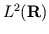means the Hilbert space of square-integrable complex function on the real line. If we define the unitary operators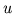and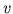ongiven by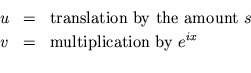we can see that they don't commute, but instead satisfywhere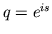. (Note: I didn't say which way to translate by the amount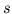-- if you pick this correctly things will work out.)

The algebra of operators ongenerated byandand their inverses is called the noncommutative torus. (If you know how, it's better to take the C*-algebra generated by two unitariesandsatisfyingThis is actually quite a bit bigger for.) This is clearly a natural sort of thing because it's built up out of simple translation and multiplication operators, and all of Fourier theory is based on the interplay between translation and multiplication operators.

Why is called a "torus"? Note that it depends on the parameter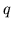. If we take,is the C*-algebra generated by two unitariesandthat commute. This may identified the algebra of functions on a torus if we think ofas multiplication byandas multiplication by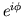, where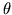and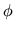are the two angles on the torus. So we've got a one-parameter family of algebrassuch that when, it's just the algebra of (continuous) functions on a torus, but fornot equal to one we have some sort of noncommutative analog thereof. The parametermeasures noncommutativity or "quantum-ness", and one can relate it to Planck's constant (which also measures "quantum-ness") byThis example is actually the tip of an iceberg called called "deformation theory". One can read more about it in these papers, among others:
• M. Rieffels, Deformation quantization and operator algbras, Proc. Symp. Pure Math. 51 (1990), 411-423.
• Bayen, Flato, Fronsdal, Lichnerowicz and Sternheimer, Deformation theory and quantization, Ann. Physics 111 (1978), 61-151.
As you can see from these titles, it has a lot to do with quantization, since in quantization one is trying to start with a commutative algebra of functions (observables) on phase space and "quantize" it, or make it noncommutative, somehow.

Now it shouldn't be too surprising that the noncommutative torus is an r-commutative algebra, since the commutation relations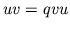tell you exactly how to "switch"and. I've shown that there is a unique way to make the noncommutative torus into a strong r-commutative algebra such that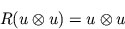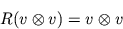and(Recall that "strong" simply means thatis the identity, so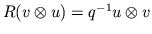.) One may thus go ahead and define "r-commutative differential forms" for the noncommutative torus, which satisfyand more relations obtained by differentiating these. One can then calculate the (r-commutative) de Rham cohomology of the noncommutative torus, and, lo and behold, it's isomorphic to that of the usual torus. This fits into the philosophy that the noncommutative torus is obtained from the usual torus by a "continuous deformation" -- no holes have been formed or gotten rid of.

If you're interested in learning more about noncommutative tori, a good review article is

• M. Rieffel, Noncommutative tori: a case study of noncommutative differential manifolds, in Geometric and topological invariants of elliptic operators Contemp. Math. 105, American Mathematical Society, 1990, pp. 191-211.

Let me just conclude by saying that noncommutative tori have applications in physics. This shouldn't really be surprising since they're such simple things. Let's say you have a charged particle trapped on the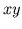plane, and there's a magnetic field of constant intensity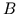perpendicular to the plane. Then the momentum operators in the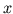direction and thedirection no longer commute. Exponentials of these (i.e., translations) generate a noncommutative torus, and this fact has been used by Bellisard to do certain calculations of the quantum Hall effect! See:

• J. Bellisard, K-theory of C*-algebras in solid state physics, in Lecture Notes in Physics vol. 237, Springer, Berlin, 1986, pp. 99-156.

Next: Charged Particles in a Magnetic Field Up: Braids and Quantization Previous: r-Algebras